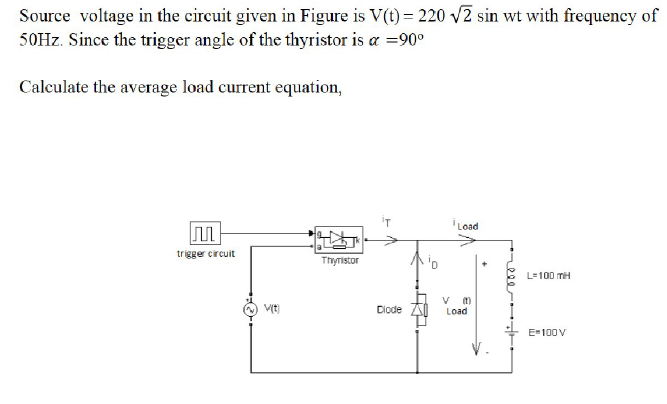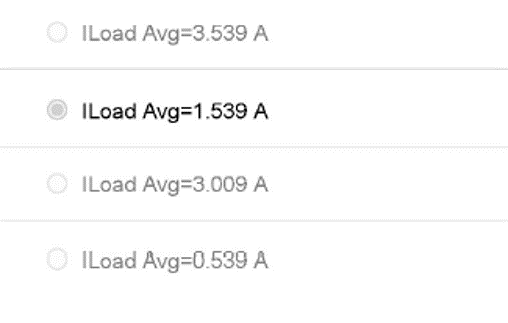# Question Source voltage in the circuit given in Figure is V(t)= 220 V2 sin wt with frequency of 50Hz. Since the trigger angle of the thyristor is a =90° Calculate the average load current equation, Load trigger circuit Thyristor L=100 MH Wit Diode Load E=100V ILoad Avg=3.539 A ILoad Avg=1.539 A ILoad Avg=3.009 A ILoad Avg=0.539 ACRHOWG The Asker · Electrical EngineeringTranscribed Image Text: Source voltage in the circuit given in Figure is V(t)= 220 V2 sin wt with frequency of 50Hz. Since the trigger angle of the thyristor is a =90° Calculate the average load current equation, Load trigger circuit Thyristor L=100 MH Wit Diode Load E=100V ILoad Avg=3.539 A ILoad Avg=1.539 A ILoad Avg=3.009 A ILoad Avg=0.539 A
More
Transcribed Image Text: Source voltage in the circuit given in Figure is V(t)= 220 V2 sin wt with frequency of 50Hz. Since the trigger angle of the thyristor is a =90° Calculate the average load current equation, Load trigger circuit Thyristor L=100 MH Wit Diode Load E=100V ILoad Avg=3.539 A ILoad Avg=1.539 A ILoad Avg=3.009 A ILoad Avg=0.539 A